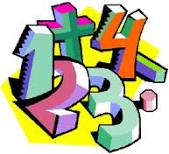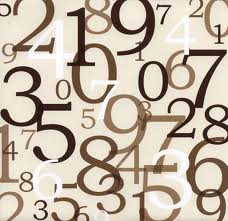# Number TheoryNumber theory explores the arithmetic of the integers, especially problems associated with divisibility. Prime numbers, having no non-trivial divisors, are especially important, yet often it is very difficult to prove theorems about them.

Diophantine equations are those where we want to find integer solutions, if there are any. The most famous of these, Fermat's Last Theorem, involves the equation xn + yn = zn. For n > 2 it has no positive solutions. Although Fermat was convinced of the truth of this statement in the 18th century it has taken till the end of the 20th century for a proof to be found, a proof moreover that requires tools beyond the borders of number theory itself.We discuss linear equations ax + b = 0 and quadratic equation ax2 + bx + c = 0 in Zn. The latter involves knowing which numbers have integer square roots in Zn.

To answer the latter question we develop the theory of quadratic residues. We define the Legendre function by: (n | m) = 0 if n is a square in Zm and 0 otherwise. By developing a large number of properties of this function, some trivial, others quite deep, we can compute (n | m) for large m far more quickly than just by squaring every element.

[Please note that all links are to Adobe .pdf documents. They will open in a separate browser window.]